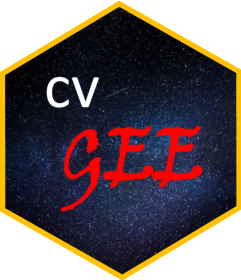## Description

cvGEE calculates cross-validated versions of the logarithmic, quadratic and spherical scoring rules based on generalized estimating equations.

The package presumes that the GEE has been solved using the geeglm() function of the geepack.

• For family = gaussian() only the quadratic rule is available calculated as the squared prediction error; lower values indicate a better predictive ability.

• For family = binomial() and dichotomous outcome data the probabilities for the two categories are calculated from the Bernoulli probability mass function. For family = binomial() and binomial data the probabilities for each possible response are calculated from a beta-binomial distribution with variance set equal to the variance from the corresponding quasi-likelihood behind the GEE. Likewise for family = poisson() the probabilities for the number of events up to a particular maximum (set using the max_count argument) are calculated using the negative binomial distribution with variance set equal to the variance from the corresponding quasi-likelihood behind the GEE. For these families all three scoring rules are available, with higher values in each rule indicating better predictive ability.

## Basic Use

We compare a linear and a nonlinear GEE for the dichotomized version of the serum bilirubin biomarker from the PBC dataset:

library("geepack")
library("cvGEE")
library("splines")
library("lattice")

pbc2$serBilirD <- as.numeric(pbc2$serBilir > 1.2)

gm1 <- geeglm(serBilirD ~ year * drug,
family = binomial(), data = pbc2, id = id,
corstr = "exchangeable")

gm2 <- geeglm(serBilirD ~ ns(year, 3, Boundary.knots = c(0, 10)) * drug,
family = binomial(), data = pbc2, id = id,
corstr = "exchangeable")

plot_data <- cv_gee(gm1, return_data = TRUE)
plot_data$linear <- plot_data$.score
plot_data\$non_linear <- unlist(cv_gee(gm2))

xyplot(linear + non_linear ~ year | .rule, data = plot_data,
type = "smooth", auto.key = TRUE, layout = c(3, 1),
scales = list(y = list(relation = "free")),
xlab = "Follow-up time (years)", ylab = "Scoring Rules")

## Installation

The development version of the package can be installed from GitHub using the devtools package:

devtools::install_github("drizopoulos/cvGEE")

and with vignettes

devtools::install_github("drizopoulos/cvGEE", build_vignettes = TRUE)